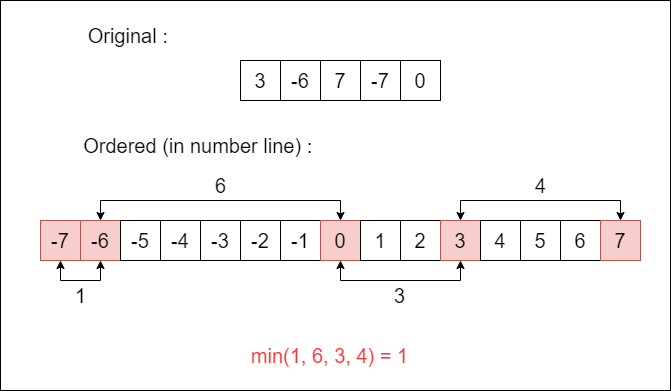# Minimum Difference in an Array

Minimum Difference in an Array.

Problem Statement: Given an array, print the minimum of the difference of all possible pairs of elements.

Examples:

```Example 1:
Input:
arr = {3,-6,7,-7,0}
Output:
Minimum Difference is 1
Explanation:
-6 and -7 give the smallest difference of all possible pairs, which is 1.

Example 2:
Input: arr = {1,6,9,12,4}
Output: Minimum Difference is 2
Explanation: 6 an 4 give the smallest difference of all possible pairs, which is 2.```

### Solution

Disclaimer: Don’t jump directly to the solution, try it out yourself first.

### Intuition:

There is no special intuition needed for this. We just need to find the minimum of all possible differences.

### Approach:

• This is a brute force approach. For each element in the array, we take a difference with all other possible elements.
• And for each such possible difference, we compare it with the global minimum difference.
• If it is smaller than the global minimum, then we update the global minimum. Else, we ignore and move on.
• We need to take absolute value of the difference, because we know that (a-b) and (b-a) are not always equal, in short subtraction is not commutative.

Code:

## C++ Code

``````#include<iostream>

#include<bits/stdc++.h>

using namespace std;

int minDiffBruteForce(int arr[], int n) {
int minDiff = INT_MAX;

for (int i = 0; i < n - 1; i++)
for (int j = i + 1; j < n; j++) {
int absDiff = abs(arr[i] - arr[j]);
minDiff = min(minDiff, absDiff);
}

return minDiff;
}

int main() {

int arr[] = {3,-6,7,-7,0};
int n = sizeof(arr) / sizeof(arr);

cout << "Minimum Difference is " << minDiffBruteForce(arr, n);

return 0;
}``````

Output: Minimum Difference is 1

Time Complexity: O(n^2), because for each element we need to calculate the difference for all other elements (nested for-loop).

Space Complexity: O(1), we are not using any extra space.

## Java Code

``````import java.util.*;

public class Solution {

public static void main(String[] args) {
int[] arr = {3,-6,7,-7,0};
int n = arr.length;

System.out.println("Minimum Difference is " + minDiffBruteForce(arr, n));
}

public static int minDiffBruteForce(int[] arr, int n) {
int minDiff = Integer.MAX_VALUE;

for (int i = 0; i < n - 1; i++)
for (int j = i + 1; j < n; j++) {
int absDiff = Math.abs(arr[i] - arr[j]);
minDiff = Math.min(minDiff, absDiff);
}

return minDiff;
}

}``````

Output: Minimum Difference is 1

Time Complexity: O(n^2), because for each element we need to calculate the difference for all other elements (nested for-loop).

Space Complexity: O(1), we are not using any extra space.

### Intuition:

• By difference, we mean the distance between two numbers in the number line. Let’s say, we have 1, -2, 2 if we plot these in the number line, the order would be -2, 1, 2.
• Now, the difference is actually the distance between them, like 1-(-2) is 3, which means we can arrive at 1 from -2 in three steps(-1, 0, 1).
• With this, we can conclude that the difference between -2 and 1 is smaller than the difference between -2 and 2 (because 2 is greater than 1).

### Approach:• From the intuition, we can say that if we order the elements, it is enough if we find the difference between adjacent elements because we need only the minimum difference of all.
• So, we first sort the elements in ascending order (Order doesn’t matter, we can also do this with descending order).
• Then, we find the differences between the adjacent elements alone. And as usual, we update the global minimum, if we find a smaller difference value.

Code:

## C++ Code

``````#include<bits/stdc++.h>

using namespace std;

int minDiffOptimal(int arr[], int n) {
sort(arr, arr + n);
int minDiff = INT_MAX;

for (int i = 1; i < n; i++)
minDiff = min(minDiff, arr[i] - arr[i - 1]);

return minDiff;
}

int main() {

int arr[] = {3,-6,7,-7,0};
int n = sizeof(arr) / sizeof(arr);

cout << "Minimum Difference is " << minDiffOptimal(arr, n);

return 0;
}``````

Output: Minimum Difference is 1

Time Complexity: O(nlog(n) + n)  ~ O(nlog(n)), because we have sorted the elements first and visited each element exactly once.

Space Complexity: O(1), we are not using any extra space.

## Java Code

``````import java.util.*;

public class Solution {

public static void main(String[] args) {
int[] arr = {3,-6,7,-7,0};
int n = arr.length;

System.out.println("Minimum Difference is " + minDiffOptimal(arr, n));
}

public static int minDiffOptimal(int[] arr, int n) {
Arrays.sort(arr);
int minDiff = Integer.MAX_VALUE;

for (int i = 1; i < n; i++)
minDiff = Math.min(minDiff, arr[i] - arr[i - 1]);

return minDiff;
}

}``````

Output: Minimum Difference is 1

Time Complexity: O(nlog(n) + n)  ~ O(nlog(n)), because we have sorted the elements first and visited each element exactly once.

Space Complexity: O(1), we are not using any extra space.

Special thanks to Prathap P for contributing to this article on takeUforward. If you also wish to share your knowledge with the takeUforward fam, please check out this article